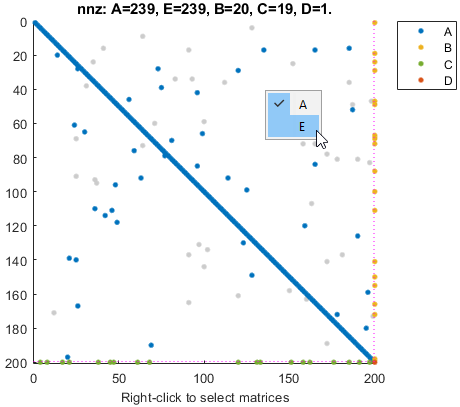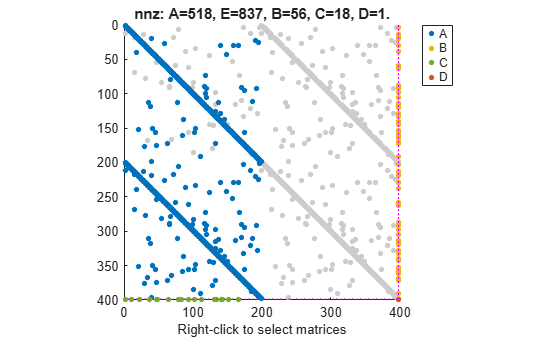# spy

Visualize sparsity pattern of a sparse model

Since R2020b

## Syntax

``spy(sys)``
``spy(AX,sys)``

## Description

example

````spy(sys)` plots the sparsity pattern of the sparse model `sys`, which can be a first-order (`sparss`) or second-order (`mechss`) model. The plot displays the number of nonzero elements in each sparse matrix of `sys`. To display a matrix, right click the plot and select the desired matrix.```
````spy(AX,sys)` plots the sparsity pattern on the `Axes` or `UIAxes` object in the current figure with the handle `AX`. Use this syntax when creating apps with `spy` in the App Designer.```

## Examples

collapse all

For this example, consider `sparseFOContinuous.mat` which contains sparse matrices for a continuous-time sparse first-order state-space model.

Extract the sparse matrices from `sparseFOContinuous.mat`.

`load('sparseFOContinuous.mat','A','B','C','D','E');`

Create the `sparss` model object.

`sys = sparss(A,B,C,D,E)`
```Sparse continuous-time state-space model with 1 outputs, 1 inputs, and 199 states. Use "spy" and "showStateInfo" to inspect model structure. Type "help sparssOptions" for available solver options for this model. ```

The output `sys` is a continuous-time `sparss` model object containing with 199 states, 1 input and 1 output.

You can use the `spy` command to visualize the sparsity of the `sparss` model object.

`spy(sys)`For this example, consider `sparseFODiscrete.mat` which contains sparse matrices for a discrete-time sparse first-order state-space model.

Extract the sparse matrices from `sparseFODiscrete.mat`.

`load('sparseFODiscrete.mat','A','B','C','D','E','ts');`

Create the `sparss` model object.

`sys = sparss(A,B,C,D,E,ts)`
```Sparse discrete-time state-space model with 1 outputs, 1 inputs, and 398 states. Use "spy" and "showStateInfo" to inspect model structure. Type "help sparssOptions" for available solver options for this model. ```

The output `sys` is a discrete-time `sparss` model object containing with 398 states, 1 input and 1 output.

You can use the `spy` command to visualize the sparsity of the `sparss` model object.

`spy(sys)`You can also view model properties of the sparss model object.

`properties('sparss')`
```Properties for class sparss: A B C D E Scaled StateInfo SolverOptions InternalDelay InputDelay OutputDelay InputName InputUnit InputGroup OutputName OutputUnit OutputGroup Notes UserData Name Ts TimeUnit SamplingGrid ```

For this example, consider the sparse matrices for the 3-D beam model subjected to an impulsive point load at its tip in the file `sparseBeam.mat`.

Extract the sparse matrices from `sparseBeam.mat`.

`load('sparseBeam.mat','M','K','B','F','G','D');`

Create the `mechss` model object by specifying `[]` for matrix `C`, since there is no damping.

`sys = mechss(M,[],K,B,F,G,D)`
```Sparse continuous-time second-order model with 3 outputs, 1 inputs, and 3408 degrees of freedom. Use "spy" and "showStateInfo" to inspect model structure. Type "help mechssOptions" for available solver options for this model. ```

The output `sys` is a `mechss` model object containing a 3-by-1 array of sparse models with 3408 degrees of freedom, 1 input, and 3 outputs.

You can use the `spy` command to visualize the sparsity of the `mechss` model object.

`spy(sys)`For this example, consider the sparse matrices of the discrete system in the file `discreteSOSparse.mat`.

Load the sparse matrices from `discreteSOSparse.mat`.

`load('discreteSOSparse.mat','M','C','K','B','F','G','D','ts');`

Create the discrete-time `mechss` model object by specifying the sample time `ts`.

`sys = mechss(M,C,K,B,F,G,D,ts)`
```Sparse discrete-time second-order model with 1 outputs, 1 inputs, and 28408 degrees of freedom. Use "spy" and "showStateInfo" to inspect model structure. Type "help mechssOptions" for available solver options for this model. ```

The output `sys` is a discrete-time `mechss` model object with 28408 degrees of freedom, 1 input, and 1 output.

You can use the `spy` command to visualize the sparsity pattern of the `mechss` model object. You can right-click on the plot to select matrices to be displayed.

`spy(sys)`## Input Arguments

collapse all

Sparse model, specified as a one of the following objects.

When `sys` contains interfaced or interconnected components, use `spy(xsort(sys))` to view the underlying block arrow structure. For more information about the block arrow structure, see `xsort`.

Object handle, specified as an `Axes` or `UIAxes` object. Use `AX` to create apps with `spy` in the App Designer.

## Version History

Introduced in R2020b